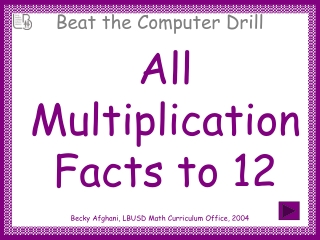Download PresentationAll Multiplication Facts to 12

# All Multiplication Facts to 12

Download Presentation## All Multiplication Facts to 12

- - - - - - - - - - - - - - - - - - - - - - - - - - - E N D - - - - - - - - - - - - - - - - - - - - - - - - - - -
##### Presentation Transcript

1. All Multiplication Facts to 12 Becky Afghani, LBUSD Math Curriculum Office, 2004

2. A slide will appear with a problem. • Say the answer aloud before the computer can answer (3 sec). • You will hear a sound when the slide changes. Directions

3. 3 x 0 =

4. 3 x 0 = 0

5. 4 x 3 =

6. 4 x 3 = 12

7. 7 x 1 =

8. 7 x 1 = 7

9. 6 x 6 =

10. 6 x 6 = 36

11. 10 x 0 =

12. 10 x 0 = 0

13. 10 x 3 =

14. 10 x 3 = 30

15. 7 x 5 =

16. 7 x 5 = 35

17. 4 x 2 =

18. 4 x 2 = 8

19. 9 x 4 =

20. 9 x 4 = 36

21. 7 x 6 =

22. 7 x 6 = 42

23. 4 x 4 =

24. 4 x 4 = 16

25. 4 x 0 =

26. 4 x 0 = 0

27. 12 x 2 =

28. 12 x 2 = 24

29. 10 x 2 =

30. 10 x 2 = 20

31. 7 x 2 =

32. 7 x 2 = 14

33. 6 x 4 =

34. 6 x 4 = 24

35. 12 x 7 =

36. 12 x 7 = 84

37. 10 x 5 =

38. 10 x 5 = 50

39. 8 x 1 =

40. 8 x 1 = 8

41. 5 x 5 =

42. 5 x 5 = 25

43. 12 x 6 =

44. 12 x 6 = 72

45. 10 x 6 =

46. 10 x 6 = 60

47. 9 x 7 =

48. 9 x 7 = 63

49. 3 x 2 =

50. 3 x 2 = 6